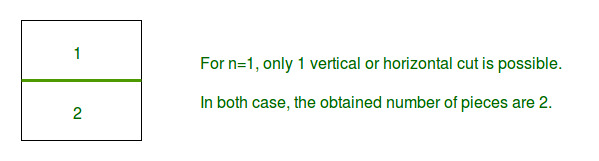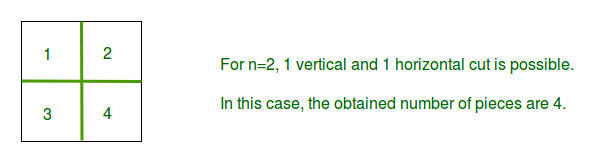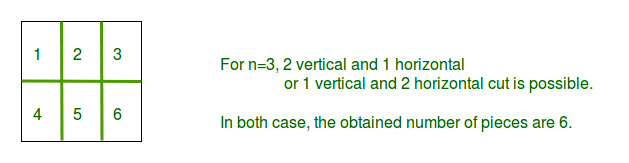Related Articles

# Maximum number of pieces in N cuts

• Difficulty Level : Medium
• Last Updated : 20 Apr, 2021

Given a square piece and a total number of cuts available n, Find out the maximum number of rectangular or square pieces of equal size that can be obtained with n cuts. The allowed cuts are horizontal and vertical cut.
Note: Stacking and folding is not allowed.
Examples

```Input : n = 1
Output : 2
Explanation : ``````Input : n = 2
Output : 4
Explanation : ``````Input : n = 3
Output : 6
Explanation : ```` `

Given is n which is the number of allowed cuts. As it is required to maximize number of pieces after n cuts, So number of horizontal cuts will be equal to number of vertical cuts. This can be prove using differentiation. So number of horizontal cut will be n/2. and vertical cuts will be n-n/2.
So number of pieces = (horizontal cut + 1) * (vertical cut + 1).
Program:

## C++

 `// C++ program to find maximum no of pieces``// by given number of cuts``#include ``using` `namespace` `std;` `// Function for finding maximum pieces``// with n cuts.``int` `findMaximumPieces(``int` `n)``{``    ``// to maximize number of pieces``    ``// x is the horizontal cuts``    ``int` `x = n / 2;` `    ``// Now (x) is the horizontal cuts``    ``// and (n-x) is vertical cuts, then``    ``// maximum number of pieces = (x+1)*(n-x+1)``    ``return` `((x + 1) * (n - x + 1));``}` `// Driver code``int` `main()``{` `    ``// Taking the maximum number of cuts allowed as 3``    ``int` `n = 3;` `    ``// Finding and printing the max number of pieces``    ``cout << ``"Max number of pieces for n = "` `<< n``         ``<< ``" is "` `<< findMaximumPieces(3);` `    ``return` `0;``}`

## Java

 `// Java program to find maximum``// no of pieces by given number``// of cuts``import` `java.util.*;` `class` `GFG``{``// Function for finding maximum``// pieces with n cuts.``public` `static` `int` `findMaximumPieces(``int` `n)``{``    ``// to maximize number of pieces``    ``// x is the horizontal cuts``    ``int` `x = n / ``2``;` `    ``// Now (x) is the horizontal cuts``    ``// and (n-x) is vertical cuts, then``    ``// maximum number of pieces = (x+1)*(n-x+1)``    ``return` `((x + ``1``) * (n - x + ``1``));``}` `// Driver code``public` `static` `void` `main (String[] args)``{``    ``// Taking the maximum number``    ``// of cuts allowed as 3``    ``int` `n = ``3``;``    ` `    ``// Finding and printing the``    ``// max number of pieces``    ``System.out.print(``"Max number of pieces for n = "` `+``                   ``n + ``" is "` `+ findMaximumPieces(``3``));``        ` `}``}` `// This code is contributed by Kirti_Mangal`

## Python 3

 `# Python 3 program to find maximum no of pieces``# by given number of cuts`` ` `# Function for finding maximum pieces``# with n cuts.``def` `findMaximumPieces(n):` `    ``# to maximize number of pieces``    ``# x is the horizontal cuts``    ``x ``=` `n ``/``/` `2`` ` `    ``# Now (x) is the horizontal cuts``    ``# and (n-x) is vertical cuts, then``    ``# maximum number of pieces = (x+1)*(n-x+1)``    ``return` `((x ``+` `1``) ``*` `(n ``-` `x ``+` `1``))`` ` `# Driver code``if` `__name__ ``=``=` `"__main__"``:`` ` `    ``#Taking the maximum number of cuts allowed as 3``    ``n ``=` `3`` ` `    ``# Finding and printing the max number of pieces``    ``print``(``"Max number of pieces for n = "` `+``str``( n)``         ``+``" is "` `+` `str``(findMaximumPieces(``3``)))` `# This code is contributed by ChitraNayal`

## C#

 `// C# program to find maximum``// no of pieces by given number``// of cuts``using` `System;` `class` `GFG``{` `// Function for finding maximum``// pieces with n cuts.``public` `static` `int` `findMaximumPieces(``int` `n)``{``    ``// to maximize number of pieces``    ``// x is the horizontal cuts``    ``int` `x = n / 2;` `    ``// Now (x) is the horizontal ``    ``// cuts and (n-x) is vertical``    ``// cuts, then maximum number``    ``// of pieces = (x+1)*(n-x+1)``    ``return` `((x + 1) * (n - x + 1));``}` `// Driver code``static` `public` `void` `Main ()``{``    ``// Taking the maximum number``    ``// of cuts allowed as 3``    ``int` `n = 3;``    ` `    ``// Finding and printing the``    ``// max number of pieces``    ``Console.Write(``"Max number of pieces for n = "` `+``                ``n + ``" is "` `+ findMaximumPieces(3));``}``}` `// This code is contributed by Mahadev`

## PHP

 ``

## Javascript

 ``
Output:
`Max number of pieces for n = 3 is 6`

Attention reader! All those who say programming isn’t for kids, just haven’t met the right mentors yet. Join the  Demo Class for First Step to Coding Coursespecifically designed for students of class 8 to 12.

The students will get to learn more about the world of programming in these free classes which will definitely help them in making a wise career choice in the future.

My Personal Notes arrow_drop_up